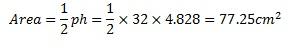# How to Find the Area of Regular Polygons

## Polygon Definition

In geometry, a polygon is a shape that is consisting of straight lines connected to create a closed loop. It also has vertices equal to the number of sides. Both of the following geometric objects are polygons.## Regular Polygon Definition

If the sides of the polygon are equal in size, and the angles are also equal, then the polygon is known as a regular polygon. Following are regular polygons.

The name of the polygons ends with suffix “gon” and the number of sides determine the front part of the name. The number in Greek is used as a prefix, and the whole word tells it is a polygon with this much sides. Following are few examples, but the list continues.

 n polygon 2 digon 3 triangle (trigon) 4 quadrilateral (tetragon) 5 pentagon 6 hexagon 7 heptagon 8 octagon 9 nonagon 10 decagon 11 hendecagon 12 Dodecagon

## How to find the area of the Polygons: Method

The area of a general irregular polygon cannot be acquired directly from the formula. However, we can separate the polygon into smaller polygons, with which we can easily calculate the area. Then, the sum of those components give the area of the whole polygon. Consider an irregular heptagon as shown below.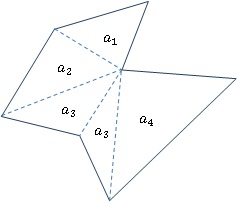The area of the heptagon can be given as the sum of the individual triangles within the heptagon. By calculating the area of the triangles (a1 through a4 ).

Total Area= a1+ a2+ a3+ a4

When the number of the sides is higher, more triangles have to be added, but the basic principle remains the same.

Using this concept, we can obtain a result for calculating the area of the regular polygons.

Consider the regular hexagon with length d sides as shown below. The hexagon can be separated into six smaller congruent triangles, and these triangles can be rearranged to from a parallelogram as shown.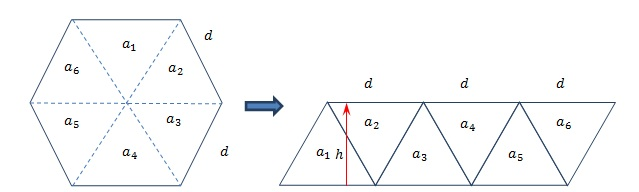From the diagram, it is clear that the sums of the area of the smaller triangles are equal to the area of the parallelogram (rhomboid). Therefore, we can determine the area of the hexagon using the area of the parallelogram (rhomboid).

Area of the parallelogram = Sum of the area of the triangles = Area of the Heptagon

If we write an expression for the area of the rhomboid, we have

AreaRhom = 3dh

By rearranging the terms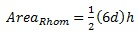From the geometry of the hexagon we can observe that 6d is the perimeter of the hexagon and h is the perpendicular distance from the center of the hexagon to the perimeter. Therefore, we can say,

Area of the hexagon=12 perimeter of hexagon ×perpendicular distance to the perimeter.

From the geometry, we can show that the result can be extended to polygons with any number of sides. Therefore, we can generalize the above expression into,

Area of the Polygon=12 perimeter of polygon ×perpendicular distance to the perimeter

The perpendicular distance to the perimeter from the center is given the name apothem (h). So, if a polygon with n sides has a perimeter p and an apothem h we can get the formula: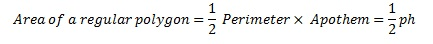## How to find the area of Regular Polygons: Example

1. An octagon has sides 4cm in length. Find the area of the Octagon. To find the area of the octagon two things are required. Those are the perimeter and apothem.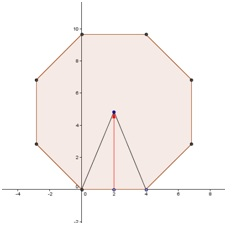• Find the perimeter

The length of a side is 4cm, and an octagon has 8 sides. Therefore, p
Perimeter of the Octagon= 4×8=32cm

• Find the Apothem.

The internal angles of the octagon are 1350 and the side of the triangle drawn bisects the angle. Therefore, we can calculate the apothem (h) using the trigonometry.

h=2tan67.50=4.828cm

• Therefore, the area of the octagon is